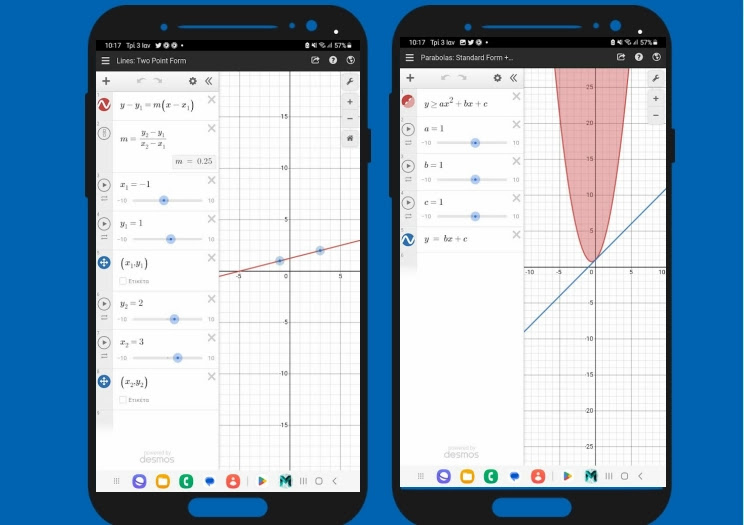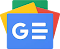## Friday April 14 2023Free application for simple as well as complex mathematical graphs, just type in the mathematical operations or equations and you will get the answers immediately. Set values ​​interactively or change any parameter to visualize its effect on the graph.

To make the app work as a scientific calculator, simply type in any you want and Desmos Graphing Calculator will show you the answer. It can handle square roots, inequalities and many other mathematical parameters.Follow us on Google News